Courses

# Detailed Chapter Notes - Atoms and Molecules, Class 9, Science | EduRev Notes

Created by: Learners Habitat

## Class 9 : Detailed Chapter Notes - Atoms and Molecules, Class 9, Science | EduRev Notes

The document Detailed Chapter Notes - Atoms and Molecules, Class 9, Science | EduRev Notes is a part of Class 9 category.
All you need of Class 9 at this link: Class 9

Atoms and molecules

Introduction

The structure of matter has been a subject of speculation from very early times. According to greek philosopher Aemocritus, if we go on dividing matter into smaller parts, a stage would be reached when particles obtained cannot be divided further. He called these particles 'atoms' meaning indivisible.

Conclusion :

All matter is made up of small particles called atoms. Different kinds of atoms and molecules have different properties due to which different kinds of matter also show different properties.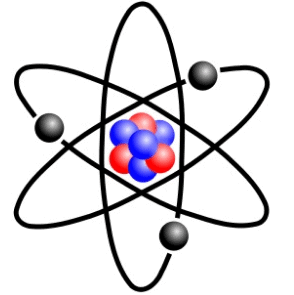Laws of chemical combination :

By studying the result of quantitative measurement of many reactions it was observed that whenever substances react, they follow certain laws. These laws are called the law of chemical combination.

(a) Law of conservation of mass.

(b) Law of constant proportions.

(c) Law of multiple proportions.

(d) Law of conservation of mass ;

This law was given by the french chemist A. Lavoisier in 1774. This law states that in every chemical reaction, the total mass before and after the reaction remains constant.

"That is mass can neither be created nor destroyed in a chemical reaction". Lavoisier showed that when mercuric oxide was heated, it produced free mercury and oxygen. The sum of masses of mercury and oxygen was found to be equal to the mass of mercuric oxide.

Mercuric oxide →  Mercury Oxygen

100 g                      92.69 g      7.49 g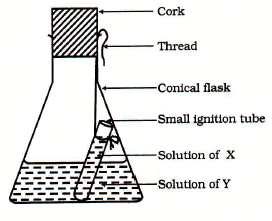Ignition tube containing solution of Z, dipped in a conical flask containing solution of Y

Activity : Demonstration of law of conservation of mass.

• Prepare seperately a 5% solution of barium chloride and a 5% solution of sodium sulphate.
• Take about 20ml of barium chloride solution in a conical flask.
• Take sodium sulphate solution in a small test tube. Hang the test tube in the conical flask with the help of a thread.
• Close the mouth of the flask with cork.
• Weigh the flask along with its contents.
• Now tilt the flask so that the two solution get mixed.
• Weigh the flask again along with its contents. What do you observe ? It is observed that on mixing the two solution a chemical reaction takes place which is indicated by the formation of a white precipitate

Barium chloride Sodium sulphate ® Barium sulphate (white ppt) Sodium Chloride

• The mass of the flask and its contents remains constant. Thus, during a chemical reaction mass is neither created nor destroyed.

This activity can also be carried out with the following pairs :

(a) Silver nitrate and sodium chloride

(b) Copper sulphate and sodium carbonate

This law was given by the french chemist A. Levoisier and Joseph Proust. This law deals with the composition of chemical compounds.

This law is : A pure chemical compound always contains same elements combined together in same proportion by mass.

For example : Pure water obtained from different sources such as river, well etc. always contains hydrogen and oxygen combined together in the ratio 1 : 8 by mass similarly carbon dioxide can be obtained by different methods such as by burning of carbon, by heating lime stone. It shows that samples of carbon dioxide obtained from different sources contain carbon and oxygen in the ratio of 3 : 8 by mass. Thus in water or CO2 this proportion of hydrogen and oxygen or carbon and oxygen always remains constant.

Ex. Hydrogen and oxygen combine in the ratio of 1 : 8 by mass to form water. What weight of oxygen gas would be required to completely react with 3 g of hydrogen gas.

Sol. Ratio in which hydrogen and oxygen combine = 1 : 8

1 g of hydrogen cmbines with oxygen = 8 g

3 g of hydrogen will combine with oxygen = 8 × 3 = 24 g.

(c) Law of multiple proportions :

It was given by Dalton in 1808. According to it, when one element combines with the other element to form two or more different compounds, the mass of one element, which combines with a constant mass of the other, bear a simple ratio to one another. Example : Carbon and oxygen when combine, can from two oxides that are CO (carbon monoxide), CO2 (carbon dioxide).

In CO, 12g carbon combine with 16g of oxygen. In CO2, 12g carbon combine with 32g of oxygen. Thus, we can see the mass of oxygen which combine with a constant mass of carbon (12g) bear simple ratio of 16 : 32 or 1 : 2.

Dalton's Atomic Theory :

On the basis of laws of chemical combination John Dalton proposed atomic theory in 1808. The main points of dalton's atomic theory are :

The main postulates of the Dalton's atomic theory are :

1.  All matter is made up of very tiny particles called atoms.
2.  Atoms are indivisible particles, which cannot be created or destroyed in a chemical reaction,
3.  Atoms of a given element are identical in mass size and chemical properties.
4. Atoms of different element have different mass size and chemical properties.
5.  Atoms combine in the ratio of small whole numbers to form compounds.
6. The relative number and kinds of atoms are constant in a given compound.

Dalton's atomic theory was based on the laws of chemical combination.

7. Atoms of same element can combine in more than one ratio to form more than one compound. For example, hydrogen and oxygen combine to give water and hydrogen peroxide. In water, two atoms of hydrogen combine with one atom of oxygen while in hydrogen peroxide, two atoms of hydrogen combine with two atoms of oxygen.

For example : The postulates of Dalton's atomic theory that "atoms can neither be created nor destroyed", was the result of law of conservation of mass and the postulates of Dalton's atomic theory that "the element consist of atom having fixed mass", and that the number and kind of atom in a given compound is fixed came from the law of constant proportions.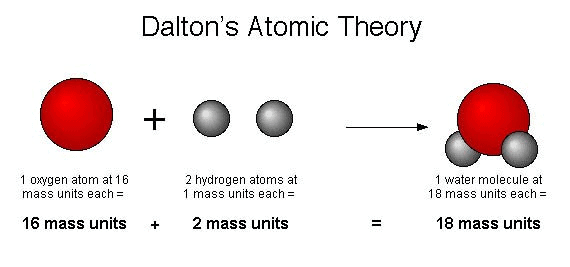Drawbacks of Dalton's Atomic Theory :

Some of the drawbacks of the Dalton's atomic theory of matter are given below :

• According to Dalton's atomic theory, atoms were thought to be indivisible. But it is now known that atoms can be further divided into still smaller particle called electrons, protons and neutrons.
• Dalton's atomic theory said that all the atoms of an element have exactly the same mass. But it is now known that atoms of the same element can have slightly different masses, as in case of isotopes.
• Dalton's atomic theory said that atoms of different elements have different masses. But it is now known that even atoms of different elements can have the same mass as in case of isobars.

Atoms

All the matter is made up of atoms. An atom is the smallest particles of an element that can take part in a chemical reaction. Atoms of most of the elements are very reactive and do not exist in the free state (as single atom). They exist in combination with the atoms of the same elements or another element. Atoms are very small in size. The size of an atom is indicated by its radius which is called atomic radius (radius of an atom). Atomic radius is measured in nanometre (nm) (1 metre = 109 nanometres or 1nm = 10_9m). Hydrogen atoms is the smallest atom of all, having an atomic radius of 0.037nm. Atoms are so small that we cannot see them under the most powerful optical microscope.

Symbol of elements :

Symbol may be defined as the abbreviation used for the name of an element. The symbol of an element are generally either the first letter or the first two letters or the first and the third letters of the name of the element. for example, the symbol of the following elements are the first letter of the name of that element.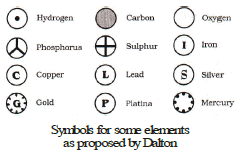first letter of the name of that element.

• Some symbols derived from the first two letters of the names of the element.

 S.No. Element Symbol 1 Hydrogen H 2 Carbon C 3 Nitrogen N 4 Oxygen O 5 Fluorine F

• Some symbol derived from the first and the third letter of the names of the elements.
 S.No. Element Symbol 1 Aluminium A1 2 Barium Ba 3 Lithium Li 4 Neon Ne 5 Calcium Ca

• There are certain symbols which seen to here no relationship to their names. The symbol of these elements are derived from their latin names.
 S.No. Element Symbol 1 Arsenic As 2 Ma gnesium Mg 3 Chlorine Cl 4 Zmc Zn 5 Chromium Cr

Atomic Mass

Atomic mass of an element may be defined as the average relative mass of an atom of the element as compared with mass of an atom of carbon (C-12 isotope) taken as 12 amu.

Atomic mass :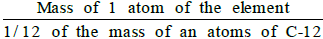How do Atoms occur :

The atoms of only a few element called noble gases (such as helium, neon, argon and krypton etc.) which are chemically unreactive and exist in the free state (as a single atom). Atoms of the elements are chemically very reactive and do not exist in the free state (as single atom).

Molecules :

A molecule is the smallest particle of an element or compound that has independent existence. A molecule contain one or more than one atoms.

The molecules of elements contain atoms of only one kind.

The number of atoms in a molecule of an element is known as atomicity of the element. For example -The atomicity of the noble gases is 1, that of hydrogen, nitrogen, oxygen etc. is 2 each and of ozone is 3. Thus, noble gases, hydrogen and ozone are respectively monoatomic, diatomic and triatomic molecules.

Molecules of elements :

The molecules of an element contain two similar atom chemically bonded together, for example ozone gas has 3 oxygen atoms combined together , so ozone exists in the form of O3. A recently discovered form of carbon, called Buckminster fullerene has molecular formula C60.

Molecules of compounds :

The molecules of a compound contain two or more different types of atoms chemically bonded together. For example : the molecule sulphur dioxide (SO2) contain one atom of sulphur chemically bonded with two atom of oxygen.

Molecular mass and formula mass :

The molecular mass of a substance (an element or a compound) may be defined as the average relative mass of a molecule of the substance as compared with mass of an atom of carbon (C-12 isotope) taken as 12 amu.

Molecular Mass =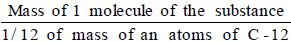The molecular mass of a compound can be obtained by adding atomic masses of all the atoms present in the molecule of the compound. For example, molecular mass of CO2 is -

12 × 1 16 × 2 = 44 u

Gram Molecular Mass :

Gram molecular mass of a substance is defined as that much quantity of the substance whose mass expressed in grams is numerically equal to its molecular mass.

For example : The molecular mass of CO2 is 44 u, its gram molecular mass is 44g. Gram molecule mass of a substance is also known as gram-molecular mass of the substance.

Formula Mass :

Formula mass of an ionic compound is obtained by adding atomic masses of all the atoms in a formula unit of the compound.

For example : Formula mass of potassium chloride (KCl)

= Atomic mass of potassium atomic mass of chlorine

39 35.5 = 74.5

Chemical formula :

The chemical formula of a compound describes the composition of a molecule of the compound in terms of the symbols of elements and the number of atoms of each element present in one molecule of the compound.

• In the chemical formula of a compound, the elements present are denoted by their symbols and the number of atoms of each element are denoted by writing their number as subscripts to the symbols of the respective element.

Example : Water is a compound whose one molecule is made up of two atoms of hydrogen and one atom of oxygen and hence its chemical formula is H2O.

• While writing the formula of an ionic compound the metal is written on the left hand side while the non-metal is written on the right hand side. The name of the metal remains as such but that of the non-metal is changed to have the ending 'ide'.

Example : MgO is named as magnesium oxide, KCl is named potassium chloride etc.

• Molecular compounds, formed by the combination between two different non-metals, are written in such a way that the less electronegative element is written on the left hand side while the more electronegative element is written on the right hand side. In naming molecular compounds, the name of the less negative non-metal is written as such but the name of the more electronegative element is changed to have the ending 'ide'.

Example : H2S is named as hydrogen sulphide.

• When there are more than one atoms of an element are present in the formula of the compound, then the number of atoms are indicated by the use of appropriate prefixes (Mono for : 1, di for 2, tri for 3. tetra for 4 atoms etc.) in the name of the compound.

Example : CO2 is named as carbon di oxide, CCl4 is named as carbon tetra chloride.

The prefixes are needed in naming those binary compounds in which the two non-metals form more than one compounds (by having different number of atoms).

Example : Two non-metal, nitrogen and oxygen, combine to form different compound like nitrogen monoxide (NO), nitrogen di-oxide (NO2), Nitrogen tri oxide (N2O3) etc.

l But, if two non-metals form only one compound, then prefixes are not used in naming such compounds.

Example : Hydrogen and sulphur combine to form only one compound H2S, So, H2S is named as hydrogen sulphide and not hydrogen monosulphide.

Ions : An ion is a positively or negatively charged atom (or group of atoms)

These are two type of ions :

(1) cations  (2) anions

(1) Cations : A positively charged ion is known as cation. For example : Sodium ion:Na , Magnesium ion: Mg2

A cation is formed by the loss of one or more electrons by an atom

For example : sodium atom, loses one electron to form a sodium ion Na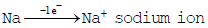Sodium atom    (A cation)

(2) Anions : A negatively charged ion is known as anion. Cl- (chloride ion), O-2 (oxide ion) etc.

An anions is formed by the gain of one or more electrons by an atom. For example a chlorine atom gains one electron to form a chloride ion Cl-.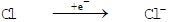Chlorine atom   Chloride ion (An anion)

Valency of ions : The valency of an ion is same as the Charge present on the ion.

Monovalent cation (Valency of cation 1)

Example : Sodium ion (Na ). Potassium ion (K ), Hydrogen ion (H ).

Divalent cations (valency of cations 2)

Example : Magnesium ion (Mg 2) Ferrous ion (Fe 2)

Trivalent cations (valency of cations 3)

Example : Aluminium ion (Al 3), Ferric ion (Fe 3).

Monovalent anion (anion of valency -1)

Example : Chloride ion (Cl-), Bromide ion (Br-)

Divalent anions (Anions of Valency _2)

Example : Oxide ion (O_2), Peroxide ion (O2_2) etc.

Trivalent anion (anions of valency -3)

Example : Nitride ion (N-3), Phosphate ion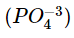etc.

Writing of formula of Molecular compound :

Steps : . The steps to be followed for writing the formula of molecular compound are-

• First, write the symbols of the elements contributing in the compound.
• Then, below each symbol, write its corresponding valency
• Finally, we exchange the valencies of the combining atoms that is with first atom, we write the valency of the second atom and with second atom, we write the valency of the first atom, the valencies to be written as subscripts to the symbols.
• If the valencies have any common factor, then the formula is divided by the common factor. This gives the required formula of the compound.

Example : To work out the formula of hydrogen sulphide

(1) Hydrogen sulphide compound is made up of hydrogen and sulphur elements. So first we write down the symbol of hydrogen and sulphur.

(2) The valency of hydrogen is 1 and the valency of sulphur is 2. So below the symbol H we write 1 and below the symbol S we write 2.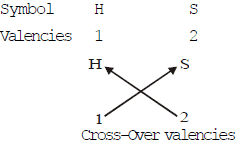Cross-Over valencies

• We now cross-over the valencies of H and S atoms. With H atom we write the valency of S (which is 2) so that it becomes H2 with S atom we write the valency of H (which is 1) so that it becomes S1. Now, joining together H2 and S1 the formula of hydrogen sulphide becomes H2S1 or H2S (This is because we don't write the subscript 1 with an atom in a formula).

Writing the formula of Ionic compound :

Steps :

• First, write the symbols of the ions from which the ionic compound is made. As a convention, the cation is written on the left side while the anion is written on the right side.
• Then, the valencies of the respective cation and anion are written below their symbols.
• The valencies of the cation and anion are exchanged. The number of cation and anions in the formula of the compound are adjusted in such a way that total positive charge of cation become equal to the total negative charge of the anions making the ionic compound electrically neutral.
• The final formula of the ionic compound is then written but the charges present on the cation and the anion are not shown.

Example : To write the formula for sodium carbonate.

(1) First, write the symbol of sodium ion and carbonate ion and write their valencies below their symbols are shown.

Symbols       Na         CO3

Valencies     1           -2

(or charges)

(2) Now, we exchange the valencies of sodium ion and carbonate ion,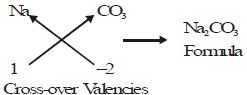(3) So  -2 gets associated with Na and 1 gets associated with CO3 in this way we get Na2 and CO3 and final formula of sodium carbonate is Na2CO3.

 Name of the compound Positive ion (cation) Negative ion (anion) Chemical Formula Name Formula Valency number Name Formula Valency number Hydrogen chloride Hydrogen H 1 Chloride Cl 1 HCl Hydrogen sulphide Hydrogen H 1 Sulphide S 2 H2S Sulphuric acid (hydrogen sulphate) Hydrogen H 1 Sulphate SO4 2 H2(SO4)1, H2(SO4) Sodium nitrate Sodium Na 1 Nitrate NO3 1 Na1(NO3)1, NaNO3 Aluminium Phosphate Aluminium A1 3 Phosp hate PO4 3 Al3(PO4)3, AIOP4 Aluminium sulphate Aluminium A1 3 Sulphate SO4 2 Al2(SO4)3 Ferrous sulphate Ferrous Fe 2 Sulphate SO4 2 Fe2(SO)2, FeSO4 Ferric sulphate Ferric Fe 3 Sulphate SO4 2 Fe2(SO4)3 Potassium dichromate Potassium K 1 Dichromate Cr2O7 2 K2(Cr2O7)1, K2Cr2O7 Magnesium nitrate Ma gnesium Mg 2 N itrate NO3 1 Mg(NO3)2 Silver chromate Silver Ag 1 Chromate Cr2O4 2 Ag2CrO4 Barium carbonate Barium Ba 2 Carbonate CO3 2 Ba2(CO3)2, BaCO3 Potassium permanganate Pota ssium K 1 Perm a nganate MnO4 1 KMnO4 Calcium hydroxide Calcium Ca 2 Hydroxide OH 1 Ca (OH)2 Aluminium oxide Aluminium A1 3 Oxide O 2 Al2O3 Magnesium phosphate Me gnesium Mg 2 Phosphate PO4 3 Mg3(PO4)2 Ammonium sulphate Am monium NH4 1 Sulphite SO3 2 (NH4)2SO3 Zinc phosphate Zinc Zn 2 Phosphate PO4 3 Zn3(PO4)2

 - 1 Charge - 2 Charge - 3 Charge Fluoride F- Sulphide S2- Phosphide P3- Chloride Cl- Oxide O2- Nitride N3- Bromide Br- Iodide I- Hydride H- ____________________ .■

Mole Concept :

Mole : Mole is a link between the mass of atoms (or molecules) and the number of atoms (or molecules). A group of 6.022 × 1023 particles (atom, molecules or ions) of a substance is called a mole of that substance.
Thus , 1 mole of atoms = 6.022 × 1023 atoms.

1 mole of molecules = 6.022 × 1023 molecules.

For example : oxygen atom in O and oxygen molecule is O2.
1 mole of oxygen atoms (O) = 6.022 × 1023 oxygen atom
1 mole of oxygen molecules = 6.022 × 1023 oxygen molecules.
Number of 6.022 × 1023, which represents a mole is known as Avogadro number.

Moles of Atoms : One mole of atoms of an element has a mass equal to the gram atomic mass of the element.

1 mole of atoms of an element = Gram atomic mass of the element.

For example : The atomic mass of oxygen (O) is 16 u, so gram atomic mass of oxygen will be 16 gram.
1 mole of oxygen atoms = Gram atomic mass of oxygen = 16 gram.

Mole of Molecules :

1 mole of molecules of an substance has mass equal to the gram molecular mass of the substance.
1 mole of molecules of a substance = Gram molecular mass of the substance.

For example : The molecular mass of oxygen (O2) is 32 u. So the gram molecular mass of oxygen molecule is 32 grams.
1 mole of oxygen molecules = Gram molecular mass of oxygen = 32 gram.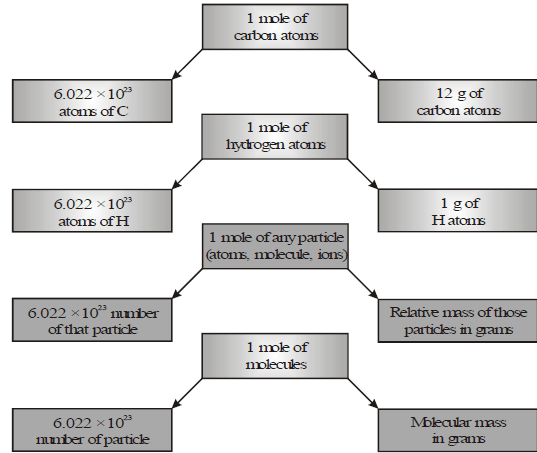Relationship between mole, Avogardro nunfoer and mass

 Atomic masses of some common elements (in amuoru) S .No. Ele me lit Symbol Atomic mass S .No. Element Symbol Atomic mass 1 Hydorgen H 1 14 Sulphur S 32 2 Helium He 4 15 Chlorine Cl 35.5 3 Lithium Li 7 16 Argon Ar 40 4 Boron B 11 17 Potassium K 39 5 Carbon C 12 18 Calcium Ca 40 6 Nitrogen N 14 19 Iron Fe 56 7 Oxygen O 16 20 Copper Cu 63.5 8 Fhiorine F 19 21 Zinc Zn 65 9 Neon Ne 20 22 Silver Ag 108 10 Sodium Na 23 23 Platinum Pt 195 11 Magnesium Mg 24 24 Gold An 197 12 Aluminium Al 27 25 Lead Pb 207 13 Phosphorus P 31 26 Uranium U 238

Mass percentage of an element from molecular formula :

The molecular formula of a compound may be defined as the formula which specifies the number of atoms of various element in the molecule of the compound.

For example : The molecular formula of glucose is C6H12O6. This shows that a molecule of glucose contains six atoms of carbon, twelve atoms of hydrogen and six atoms of oxygen. With the help of molecular formula of a compound we can calculate its percentage composition by mass. First we calculate the molecular mass of the compound. From this we can find out mass of one mole of the compound, which is equal to its gram molecular mass. Then we calculate mass of each element in one mole of the compound. The mass percentage of each element is then calculated by the following formula.

Mass percentage of element X =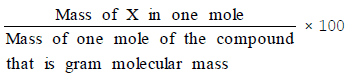Determination of Molecular formula :

To find out the molecular formula of a compound, the first is to determine its empirical formula from the percentage composition. The empirical formula of a compound may be defined as the formula which gives the simplest whole number ratio of atoms of the various elements present in the molecule of the compound.

For example : The empirical formula of the compound glucose (C6H12O6) is CH2O which shows that C, H and O are present in the simplest ratio of 1 : 2 : 1.

Molecular formula is whole number multiple of empirical formula thus,

Molecular formula = Empirical formula × n

n =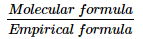Where n = 1, 2, 3.........

n =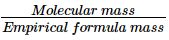Steps for writing an empirical formula :

The percentage of the element in the compound is determined by suitable methods and from the data collected, the empirical formula is determined by the following steps-

• Divide the percentage of each element by its atomic mass. This gives the relative number of moles of various elements present in the compound.
• Divide the quotients obtained in the above step by the smallest of them so as to get a simple ratio of moles of various elements.
• Multiply the figures, so obtained by a suitable integer, if necessary, in order to obtain whole number ratio.
• Finally write down the symbols of the various elements side by side and put the above number as the subscripts to the lower right hand corner of each symbol. This will represent the empirical formula of the compound.

Steps for writing the molecular formula :

• Calculate the empirical formula as describe above.
• Find out the empirical formula mass by adding the atomic masses of all the atoms present in the empirical formula of the compound.
• Divide the molecular mass (determined experimentally by some suitable method) by the empirical formula mass and find out the value of "n"
• Multiply the empirical formula of the compound with n so as to find out the molecular formula of the compound.

,

,

,

,

,

,

,

,

,

,

,

,

,

,

,

,

,

,

,

,

,

,

,

,

,

,

,

;